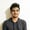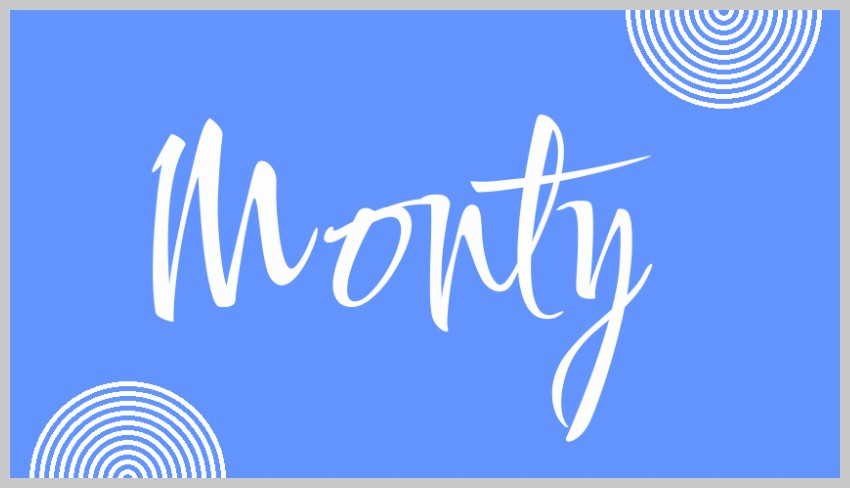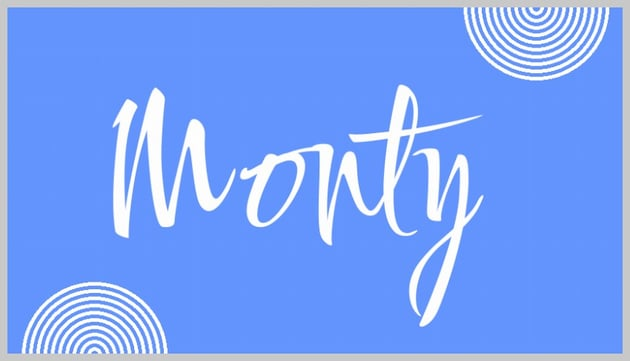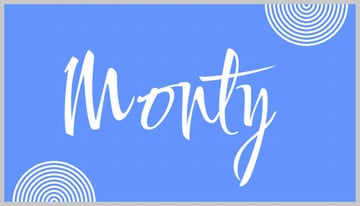# Render Text and Shapes on Images in PHPIn the previous article, we focused on loaded and manipulating images with PHP. We learned how to rotate, resize, scale or flip an image. We also learned about different filters and the convolution matrix. Those tutorials also covered some practical uses of the GD library, like resizing all images in a directory or adding watermarks on multiple images at once.

Besides using GD for manipulating regular images, we can also create our own from scratch. We can use different functions in the library to draw basic shapes like ellipses, circles, rectangles, polygons, and simple lines. With some maths, these shapes can create nice patterns. There are also functions available to draw text on the rendered image, which opens up a lot of possibilities.

This tutorial will teach you how to draw basic shapes in PHP and how to render text using your favorite font.

Here's a preview of what we'll be creating at the end of the post:## Draw Basic Shapes in PHP With GD

We will learn about basic shapes in this section and then cover line thickness, brushes, and line styles later.

You can draw a simple straight line between two given points using the imageline($image,$x1, $y1,$x2, $y2,$color) function. The $image parameter is an image resource that will have been created earlier using functions like imagecreatetruecolor() or imagecreatefromjpeg(). We will be using imagecreatetruecolor() throughout this tutorial to create new images from scratch. The function will draw a horizontal line if $y1 is equal to $y2. Similarly, it will draw a vertical line if $x1 is equal to $x2. ### Draw Circles and Arcs The function imagearc($image, $cx,$cy, $width,$height, $start,$end, $color) can draw circular arcs using $cx and $cy as its center. The $width and $height parameters determine the size of the arc on different axes. The $start and $end parameters specify the starting and ending angle of the arc in degrees. If you want to draw complete arcs from 0 to 360 degrees, you can use the alternative imageellipse($image, $cx,$cy, $width,$height, $color) function. ### Draw Rectangles and Polygons You can draw rectangles over an image using the imagerectangle($image, $x1,$y1, $x2,$y2, $color) function. The $x1 and $y1 values determine the top-left corner of the rectangle. The $x2 and $y2 values determine the bottom-right corner. There is also an imagepolygon($image, $points,$num_points, $color) function, which can create a polygon with any number of sides or points. The $points parameter is an array where two elements are paired to get the coordinates of a specific point.

Another function called imageopenpolygon() has been added to PHP 7, which does not draw a line between the first and last point.

### Putting It Together to Create a Drawing

In the following example, we have used all these functions to create a line drawing with a hut, the sun, and the ground.

The important thing here is just to figure out the value of different coordinates. I wanted to draw everything relative to the size of the original image, so I used the $img_height and $img_width variables to calculate the coordinates of different points.

## Controlling Line Thickness, Style, and Color Fills

The above image has a couple of issues, like very thin lines and no coloring. All these issues can be fixed easily using functions like imagesetthickness() and imagefilledrectangle().

The imagesetthickness($image,$thickness) function sets the thickness of rendered lines when drawing rectangles, polygons, arcs, etc. For example, setting $thickness to 5 will make any figure drawn using imagerectangle(), imagearc(), imagepolygon(), etc. 5 pixels thick. ### Drawing Filled Shapes Every drawing function also has a filled color version which fills that particular figure with a given color. For example, imagefilledrectangle() will fill the drawn rectangle with the given color. ### Using Brushes One very useful GD function is imagesetbrush($image, $brush). The $brush parameter in this function is just another image resource which can be used to draw lines. For instance, you could use a transparent vector drawing of a flower as a brush to add nice flower patterns to your image. The code snippet given below was written to use the image of a cloud as a brush when drawing a point. This adds a single cloud in our sky.

I found this cloud image on Pixabay and scaled it down to an appropriate size for our project.

The complete code for the hut image is given below. We have simply added two versions of each figure, one to draw the outline and the other to fill in the color.

This is the final result of the PHP GD code above.

## Rendering Text on Images

PHP GD comes with four different functions to let you render either multiple characters or only one character in a horizontal or vertical direction. These functions are imagechar(), imagecharup(), imagestring(), and imagestringup(). All of them accept the same six parameters, so we will just discuss the imagechar() function here.

The $font parameter imagechar($image, $font,$x, $y,$string, $color) function is simply the size of the rendered text. It only accepts integer values from 1 to 5. The $string parameter is the text that you want to render. If you pass a multi-character string to the char functions, only the first character will be rendered on the image. The imagecharup() and imagestringup() functions will render the text vertically from bottom to top.

When it comes to rendering text, the four functions we discussed above are very limited. You will find that even the largest font size value is too small for normal usage. Also, the text can only be written horizontally and vertically.

Luckily, GD also has a imagettftext($image,$size, $angle,$x, $y,$color, $fontfile,$text) function which can render the text in any font you want. The $fontfile parameter is used to specify the path to the TrueType font you want to use to display the text. The $x and \$y parameters determine the starting position of the rendered text.

The following example uses all these functions to create some nice text effects.

As you can see, we have rendered the same text with the same font in slightly different positions to create some effects like basic text shadows. The important thing to keep in mind is that the text rendered by any text function will completely hide the text below it in case of overlap. Here is the final image obtained after running the above code.

## Creating Simple Designs and Posters

Let's use all the knowledge that we have gained so far to create simple posters or images with a mix of text and shapes. I will try to recreate something similar to the image in this PHP image manipulation tutorial where we did color replacement. We will be drawing concentric circles to keep our calculations simple.

One new function that we will be using in this section is called imagettfbbox(). It basically gives us the bounding box of the text that we want to write using TrueType fonts. We will use the function to scale up our text so that either its width or height reaches a certain fraction of the image. After that, we can draw our text on the image using the imagettftext() function.

We start with a base font size of 20 points and then keep increasing it by 4 as long as both the width and height are within the defined limit. Once we reach the appropriate font size, we exit the loop and render the text on the image using imagettftext().

Now we can include the following code before the last line where we save the image to add our filled concentric circles to the bottom-left and top-right corners of the image.

As you can see, it is possible to create interesting patterns with ease using a little mathematics and some built-in PHP GD functions.

## Final Thoughts

The aim of this tutorial was to get you acquainted with different GD functions to draw basic shapes from scratch in PHP. With the help of a little maths, you will be able to use these functions to create more complicated shapes like regular polygons, rounded rectangles, etc.

PHP GD also has a couple of very useful functions for rendering text on an image. The use of a nice font will make sure that the rendered text does not look weird when placed on regular images loaded from different file paths.

Did you create any more fancy text effects in PHP? Please share them with us on the forum.